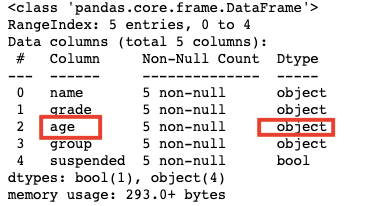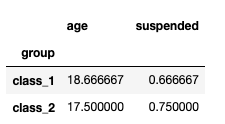Search
•Magdalena Konkiewicz

# Useful pandas functions you probably did not knowImage by AD_Images from Pixabay

Introduction

Pandas library is probably the most popular package for performing data analysis with python. There are a lot of tutorials that go through pandas basic functions but in this article, I would like to share some pandas functions that are a bit less known but can be very handy for everyday data analysis tasks.

Let's get started.

Before we get to know the functions chosen for this article we need to load some data. We will work on a data frame that we will create with the following code.

```import pandas as pd
client_dictionary = {'name': ['Michael', 'Ana', 'Sean', 'Carl', 'Bob'],
'grade': [['A', 'A'], ['C'], ['A', 'C', 'B'], [], ['F']],
'age': [19, 19, 17, 18, '-'],
'group': ['class_1', 'class_2', 'class_2', 'class_1', 'class_2'],
'suspended': [True, False, True, False, True]
}
df = pd.DataFrame(client_dictionary)```As you can see this is a very simple data frame. It represents data about students (rows) and describes their details such as names, grades, age, group, and the fact if they are suspended or not (columns).

This simple data frame will help us to demonstrate all functions that we are going to learn today.

infer_objects()

If you have been working with pandas and data frames for a while you probably have noticed that pandas are quite good at inferring data typed for columns. If you have a column with integers it will assign integer type, if they are bools it will assign boolean type, etc.

However, there are times when you will need to force it to do it. This is usually when you have a data frame with columns that were inferred as objects but with a closer look, you realize that they should be different data types.

In order to see it let's have a look at our data frame again.You can see that the column age in the last row has a dash sign instead of the number (-). This indicates probably that age for this student is unknown but causes the column to be read as an object type.

`df.info()`Let's now say that we do not want to include incomplete data so we will filter out entries that have dashes in the age column living ones that will have only integers in our case.

`df = df[df.age != '-'] `

Now let's see an info() function to see if the types of filtred data frame got updated.

`df.info()`As you can see there are fewer entries (only 4) but the type of the age column is still an object. This is when infer_objects() function can help. You can just call it on the whole data frame and it should infer the types again.

```df = df.infer_objects()
df.info()```explode()

In order to investigate explode() function, let's have a look at the data frame again.Note that column grade consists of lists of grades rather than one grade itself. There are times that you want to create an entry per item in the list rather than have the whole list as one entry. You can use explode() function to do this.

```exploded_df = df.explode('grade', ignore_index=True)
exploded_df```As you can see now there is an entry for each grade and the list 'exploded'. Note that the function explode() takes the name of the column as the parameter that is used for 'explosion'. In our case, it is 'grade'.

I have also set ignore_index parameter to True. If I have not done that each entry would have indexes from the original data frame.

pivot_table()

Pivot_table() function allows us to create pivot tables the same way as Excel would. If you have not heard of pivot tables in Excel it is probably best to learn it using Excel before trying it on pandas.

Nevertheless, pivot tables allow us to reorganize the data by specifying columns, rows, and the values that become pivot table entries. Let's have a look at our data frame again.We could use pivot_table() function to learn the average age in each group.

`pd.pivot_table(df, index='group')`You can see that we have specified the index of our pivot to be a group column. We did not specify what columns should be in the pivot table so pandas have just put there other numeric values without splitting them any further.

You can see that class_1 average age is 18.7 and 66.6% of this class is suspended, whereas class_2 average age is 17.5 and 75% of them are suspended. Pivot table entries are averages for the subgroups.

What would happen if we now specify pivot table columns to be 'suspended' column from the original data frame.

`pd.pivot_table(df, index='group', columns='suspended')`You can see that now we have a break up of ages per class and the fact if they are suspended or not. The values in the table are averages again. You can actually use a different aggregate function such as sum(), max() or min() by adding aggfunc argument.

It is important to note that columns and indexes in the pivot_table() function should be categorical variables from the original data frame. That way there will allow the split into smaller subgroups.

transpose()

Transpose() function is very simple but knowing about it is a lifesaver. It just changes columns to be rows (transposes your data frame). Its functionality is again best shown on example so let's have a look at our original data frame.And let's transpose it now.

```transposed = df.transpose()
transposed```As you can see what originally were rows, now are columns and vice versa.

Summary

In this article you have learned how to use four new functions:

• infer_objects(),

• explode(),

• pivot_table()

• and transpose()

I hope you will be able to call them whenever you need their functionality from now on. Happy coding!